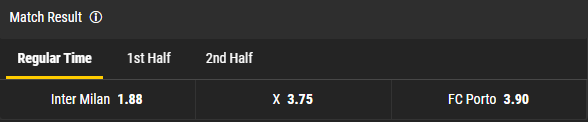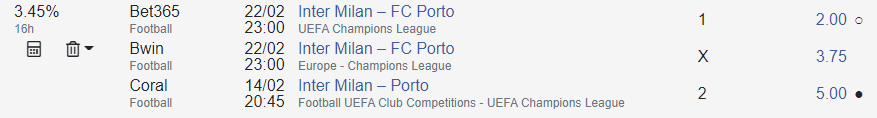# Valuebets

In this article, we will take a look at an interesting strategy known as value betting. It is more complicated than surebetting and has more risk associated with it, but it also offers certain advantages.

## What is a bookmaker’s margin?

Let us take a look at the bookmaking “kitchen”.

Imagine a coin, a usual coin with two sides: heads and tails. Assume that somebody bets that they can guess the top side of the flipped coin. It is obvious that the probability of the coin landing on either of its sides is 50/50. So it will be fair to set odds to 2 for heads and 2 for tails. That means that if two players make a bet and put a dollar on different outcomes, the winner will get 2 dollars and the loser will get nothing.

However, bookmakers never do that. In the best case scenario, a bookmaker will put 1.9 odds for heads and 1.9 for tails. If our players place their bets via a bookmaker and also bet 1 dollar each, the winner will only get \$1.90, since \$0.10 will be paid towards the bookmaker’s commission. This is called a margin. And that is how bookmakers make their living.

So in the ideal world and in a situation where a bookmaker will pay all the bet money as a prize, event odds should correlate in a certain way:

`1/K1 + 1/K2 + … + 1/Kn = 1`

Here `K1`, `K2`, … `Kn` are the odds for each of `n` opposite event outcomes – for instance, bets `1`, `X`, `2`. Each of the odds must equal to 1 divided by the probability of a corresponding outcome.

However, if we take a real line from any bookmaker and calculate the sum of probabilities, it will not equal to 1. Let us use Bwin’s odds for a Chelsea – Manchester United match as an example.`1/1.88 + 1/3.75 + 1/3.90 = 1.054` ( > 1 !!! )

`(1.054 - 1) * 100% = 5.4%` margin

Therefore, bookmakers always set their odds in a way that gives them an advantage over players. They lower the odds by factoring in their revenue. Probability is on the bookmaker’s side. That is why the majority (about 98%) of ordinary players, betting "for luck", lose their entire bank in the long run.

## What is a valuebet?

As you can see from the margin description, each bookmaker tries to guess the probability of this or that outcome and set low odds for it. It happens, however, that bookmakers make mistakes and set high odds instead.

Going back to our coin flip example, let us assume that a bookmaker decided that heads would be more likely to show and set odds of 2.1 on heads and 1.75 on tails. Clearly, the bookie is wrong. If a player knows that the probability of both outcomes is exactly 50%, he will be much better off betting on heads.

If the player places 100 bets like this one, betting a dollar each time, he will win with a 50% probability. Therefore, the player will win 100 х 50% х 2.1 = 105 dollars. With a sufficiently large number of valuebets, the player will have a 5% profit. Since the law of probability is on the player’s side in this case, his bank will grow in the long run.

To illustrate this idea, let us use another example, this time with a dice. It is apparent that the probability of any number being cast is 1 out of 6. Let two players use the following rules: the first one comes up with a number and casts the dice. If he wins, he gets 5 dollars from the other one. If he loses, he gives the other one a dollar. It is clear that the first player won’t have anything (except for a hole in the pocket) very shortly. Let us change the rules: if the first player guesses the number, he gets 7 dollars. If he does not, he will give one dollar. Now, the first player will bankrupt the second one in no time.

A valuebet is a bet that makes the following inequality true:

`K * P > 1`

where `K` is the odds and `P` is the real probability of this outcome.

## Methods of finding valuebets

To be able to find valuebets, you first need to be able to assess the real probability of event outcomes. After that, once you know the true probabilities, you need to find the odds that make the inequality `K * P > 1` true.

It is very hard to determine the true probability of outcomes in events such as a football match. Bookmakers hire entire departments of professional analysts to predict match outcomes.

There are two main methods of predicting outcomes:

• Analytical. Research of the teams’ potential, recent statistics, line-ups, evaluation of possible weather and location effects, moon phase, position of Mercury relative to Saturn, inside knowledge about fixed matches and a lot more.
• Statistical. Rely on the work of a huge army of betting analysts, consider that they are all close to reality, but the truth lies in between their opinions.

The first method is widely used by bookmakers. They try to use all the data they have to assess the chance for a certain team to win. There are professional bettors who also know the teams very well and can predict outcomes just as accurately as the bookmakers. The analytical method, however, requires extensive knowledge of the subject matter and can hardly be automated.

The second method is based on statistics. If you have the odds from a large number of bookmakers, you can calculate the bookmaker’s margin for each of the odds. You can then use the margin to try to determine how the bookmaker assessed the probability. Finally, you calculate the average value – and that will be the most accurate prediction, since it is based on the work of many analysts. This method may seem to be less accurate to some people, but it can be easily automated.

Our service uses the statistical approach for finding valuebets.

## Using surebets to find valuebets

A bookmaker’s surebet always has one interesting property. If you assume that all bets of a certain surebet belong to a single bookmaker and you calculate the margin, you will get a negative number. Let us check one of the surebets.`1/2 + 1/3.75 + 1/5 = 0.9667` ( < 1 )

Margin equals to: `(0.9667 - 1) * 100% = -3.33%`

This means that at least one of the bets in the surebet is overestimated. That is, among all bets of any surebet with a positive profit, there is at least one valuebet (but can be more). Basically, this overestimated bet has most likely originated the surebet and all other surebets are only used for backing up this one.

This way, if you have a list of found surebets for the same event, you can try to determine which bet has caused them. For example, if all surebets for the same event contain a bet for the victory of the first team by the same bookmaker, it is apparent that this very bet is overestimated.

## Pros and cons of using valuebets

Unlike surebets, valuebets have their pros and cons.